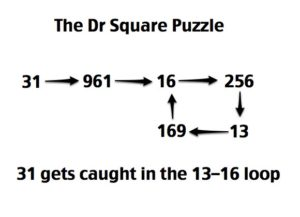# The Dr Square Puzzle Part II

November 12, 2011

Recall the puzzle:

Step 1: Choose a starting number.
Step 2: Square the number.
Step 3: Sum up the digits of that number.
Step 4: Repeat steps 2 and 3 until you understand what’s going on.

and recall that we had three discovered loops, which we called the 1 loop, the 9 loop, and the 13-16 loop. For example:I think it’s time for a few hints. Let me say, first of all, that the student and I came up with a few conjectures about this puzzle (there were also a few more that we proved were false with counterexamples).

Conjecture 1: Any number that’s a multiple of 3 ends up in the 9 loop.

Example: Thirty-nine is a multiple of three. We see that 39–>1521–>9 (and thus into the 9 loop).

Conjecture 2: It does not matter if you start by taking the digit sum or the square of your number: it ends up in the same loop either way.

I like this conjecture a lot. Let’s see why we conjectured it:

Example. First, taking the square first, we have 47–>2209–>13 (into the 13-16 loop).

Now, taking the digit sum first, we have 47–>11–>121–>4–>16 (and into the 13-16 loop)

Conjecture 3: There’s a length nine repeating pattern that determines which loop your number will end up in. In other words, you can see what loop your number ends up in by looking at its remainder after you divide by 9. (If you’re not familiar with the term, the arithmetic with remainders on division by 9 are known as mod 9 arithmetic).

Example. Take a number like 532. In mod 9, it’s the same as 1, since dividing it by 9 leaves a remainder of 1 (you can check that on your own). Therefore, it should end up in the 1 loop. Let’s see:

532–>283024–>19–>361–>10–>100–>1 (into the 1 loop)

Now we haven’t proven any of these conjectures. They’re only based on evidence we gathered from cranking out examples. However, now that we have the idea to look at mod 9 (i.e., remainders mod 9), I have some ideas/hints.

Hint 1: What is the relationship between the digit sum of a number mod 9 and the number itself?

Example. 134 is equivalent to 8 in mod 9 since 134 is 8 more than 126, which is a multiple of 9. Also, 1+3+4 = 8. Hmmm…

Hint 2: What happens when we look at squaring in mod 9?

Example. Eight squared is 64, and 64 is equivalent to 1 mod 9. But 8 also ended up in the 1 loop. Hmmm…

Hint 3: Do we really have to worry about big numbers? If you have a ten digit number, what’s the minimum number of digits the square can have? What’s the maximum number of digits the digital sum can have? Can we use this to help us focus on the part of this problem that really matters?

Solution to follow!

Subscribe
Notify of1 Comment
Inline Feedbacks